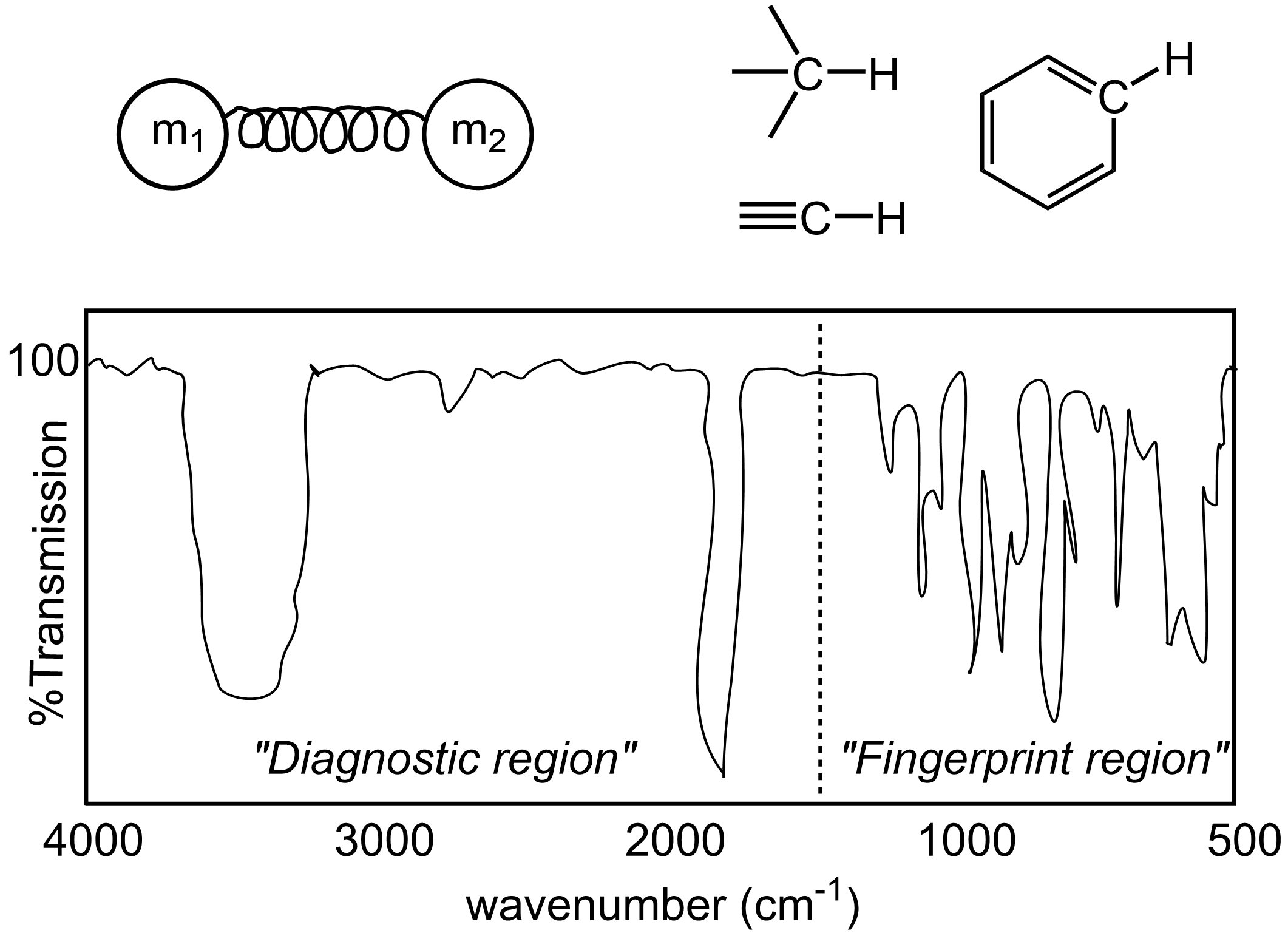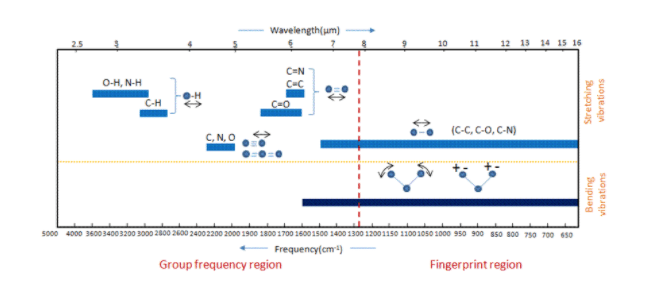MCAT CARS Strategy Course Trial Session - Tuesday at 8PM ET!

### Infrared Region

Topic: Molecular Structure And Absorption Spectra

By interpreting the frequencies of infrared light absorbed by a molecule, one can identify bond-stretching motions and therefore gain structural information such as what type of functional groups and chemical bonds are present.

Absorption of infrared radiation by an organic compound causes bonds within that molecule to be stretched, and this phenomenon can be used to gain information about the molecular structure. An analogy often used for a chemical bond between two atoms is a spring with a mass at each end. Therefore, we can model the stretching or oscillations of this bond with the equation derived from physics, ν = 1/(2π)*√(k/μ), where ν = the frequency of the oscillation (how fast the bond is stretching and contracting), k = the force constant (strength of the bond), and μ = the reduced mass of the system, m1m2/(m1+m2). From this relationship, we can further conclude that (1) a system with a larger reduced mass will have a lower stretching frequency (the spring will stretch and contract slower if it has heavier masses on each end), and (2) a system with a larger force constant will have a higher stretching frequency (a tighter-wound spring will contract faster after being stretched). To extend this to a chemical bond, consider the simplified two-body examples below.

– C-C vs. C=C
These two systems have the same reduced mass, each with two carbon atoms, but the stronger C=C double bond has a larger force constant, therefore the stretching frequency of C=C is faster/higher.
– C-C vs. C-H
In this case, the reduced mass of the C-H system, 12*1/(12+1) = 0.9, is less than that of the C-C system, 12*12/(12+12) = 6, so the higher mass C-C system has a relatively lower stretching frequency.

When an organic molecule absorbs infrared light as energy, that causes the chemical bonds to stretch (or bend or rotate depending on how much energy is put in). Therefore, shining infrared light on a sample of a compound can help diagnose the types of functional groups and bonds are in the molecule. Note that IR light has relatively longer wavelengths (is lower in energy) than UV or visible light.

Covalent bonds in organic molecules are not rigid sticks – rather, they behave more like springs.  At room temperature, organic molecules are always in motion, as their bonds stretch, bend, and twist.  These complex vibrations can be broken down mathematically into individual vibrational modes. The energy of molecular vibration is quantized rather than continuous, meaning that a molecule can only stretch and bend at certain ‘allowed’ frequencies.  If a molecule is exposed to electromagnetic radiation that matches the frequency of one of its vibrational modes,  it will in most cases absorb energy from the radiation and jump to a higher vibrational energy state – what this means is that the amplitude of the vibration will increase, but the vibrational frequency will remain the same.  The difference in energy between the two vibrational states is equal to the energy associated with the wavelength of radiation that was absorbed.  It turns out that it is the infrared region of the electromagnetic spectrum which contains frequencies corresponding to the vibrational frequencies of organic bonds.

The power of infrared spectroscopy arises from the observation that different functional groups have different characteristic absorption frequencies. Some bonds absorb infrared light more strongly than others, and some bonds do not absorb at all. In order for a vibrational mode to absorb infrared light, it must result in a periodic change in the dipole moment of the molecule.  Such vibrations are said to be infrared active. In general, the greater the polarity of the bond, the stronger its IR absorption.  The carbonyl bond is very polar, and absorbs very strongly.  The carbon-carbon triple bond in most alkynes, in contrast, is much less polar, and thus a stretching vibration does not result in a large change in the overall dipole moment of the molecule. Alkyne groups absorb rather weakly compared to carbonyls. Some kinds of vibrations are infrared inactive.  The stretching vibrations of completely symmetrical double and triple bonds, for example, do not result in a change in dipole moment, and therefore do not result in any absorption of light (but other bonds and vibrational modes in these molecules do absorb IR light).

An infrared spectrum is plotted with the x-axis giving the range of infrared frequencies in units of wavenumbers (1/wavelength; so high wavenumbers=short wavelengths=high frequencies), and the y-axis shows the %transmission at each wavenumber, giving an indication of which frequencies are absorbed by the sample (<100% transmission) and which pass through without being absorbed (close to 100% transmission). Most organic functional groups absorb in the so-called “diagnostic region” of the spectrum at wavenumbers from 1500-4000 cm-1 and can be easily recognized. For example, carbonyl functional groups (C=O) typically show up around 1750 cm-1 and hydroxyl groups (O-H) stretches show up around 3500 cm-1 (see example spectrum below). Of course, molecules are more complex than the two-body spring model, so the exact position of the peaks observed for each functional group can vary slightly depending on the molecular structure around each bond, or due to H-bonding or aromaticity (see below, all three of the C-H stretches will be unique). Some low energy bond stretches and other molecular movements such as bends and rotations of bonds absorb lower frequencies of IR radiation below 1500cm-1 in a way that is unique to each molecule, thus giving this part of the spectrum the name “fingerprint region.Practice Questions

MCAT Official Prep (AAMC)

Chemistry Question Pack Question 35

Sample Test C/P Section Passage 8 Question 40

Key Points

• Shining infrared light on an organic compound causes bonds to stretch (and bend and rotate), so by analyzing which wavelengths or energies are absorbed by a compound can help diagnose different functional groups present.

• In general, a bond between atoms with a larger reduced mass will have a lower stretching frequency (a spring stretches and contract slower if it has heavier masses on each end)

• In general, a stronger bond, i.e. double or triple bond, will have a higher stretching frequency (a tighter-wound spring will contract faster after being stretched)

Key Terms

wavenumber: x-axis frequency units for an infrared spectrum equal to 1/wavelength, so high wavenumbers=short wavelengths=high frequencies.

fingerprint region: The region between 400 cm1 and 1500 cm1 in an IR spectrum. The fingerprint region of a given compound is unique and, therefore, can be used to distinguish between compounds.

vibrational modes: The vibrational state of an oscillating system in which the frequency of vibration is the same for all elements.

infrared active: A molecule or substance absorbs some frequency in the infrared spectrum.

infrared inactive: When no change in dipole moment during the symmetric stretch vibration and the 1340 cm-1 band is not observed in the infrared absorption spectrum of a molecule or substance.

Billing Information